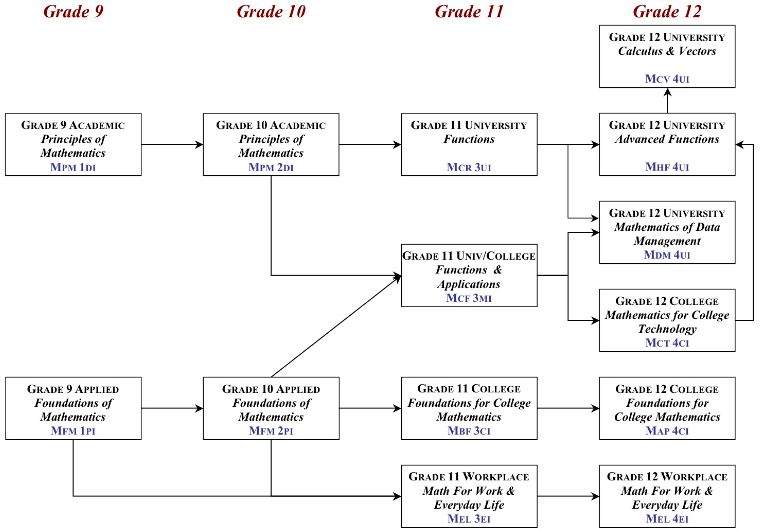# MATH Tutors

### MATH & ME

Students will build a strong mathematical foundation by being introduced to the five major strands of the Ontario math curriculum. They will learn how to add, subtract, tell time, count money, and sort and classify shapes and figures.

### STEPPING UP

Students will build on their existing knowledge math by solving basic multiplication and division problems, examining areas of rectangles and parallelograms, and exploring fractions, money, decimals, and integers.

### MASTERMINDS